Question

1) In the laws of optics experiment, a student measured the intensity of a light source at different distances with respect to the light source using a light probe. The following table shows the data collected in this experiment.

 r (m) I (Lux) 0.050 636.6 0.100 159.2 0.150 70.7 0.200 39.8 0.250 25.5 0.300 17.7 0.350 13.0 0.400 9.9 0.450 7.9 0.500 6.4

Plot the data using excel and apply the power law fit (y(x) = A x-n). What is the value of n?

2) In Question 1, what is the value of A from the fitting equation?

3) Use your findings in the previous question and the data in Question 1 to determine the intensity at r = 0.521 m.

4) Use your findings in previous questions and the data in Question 1 to determine the distance when the intensity is 117.1 Lux.

5) In the law of refraction experiment, a student measured the incident angle in air (65.6o) and the refracted angle in water (39.9o). If the index of refraction for air is 1.00, then calculate the medium index of refraction.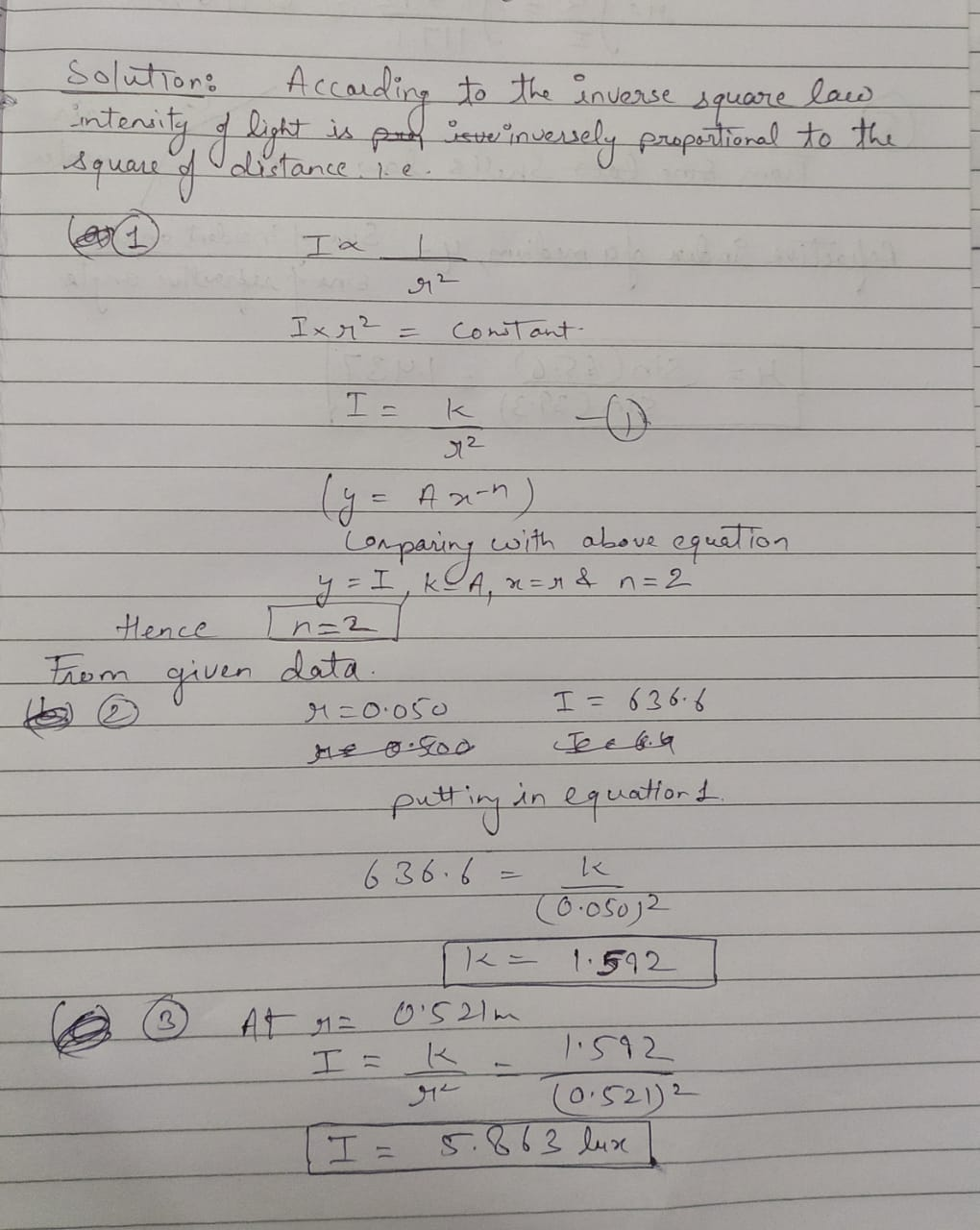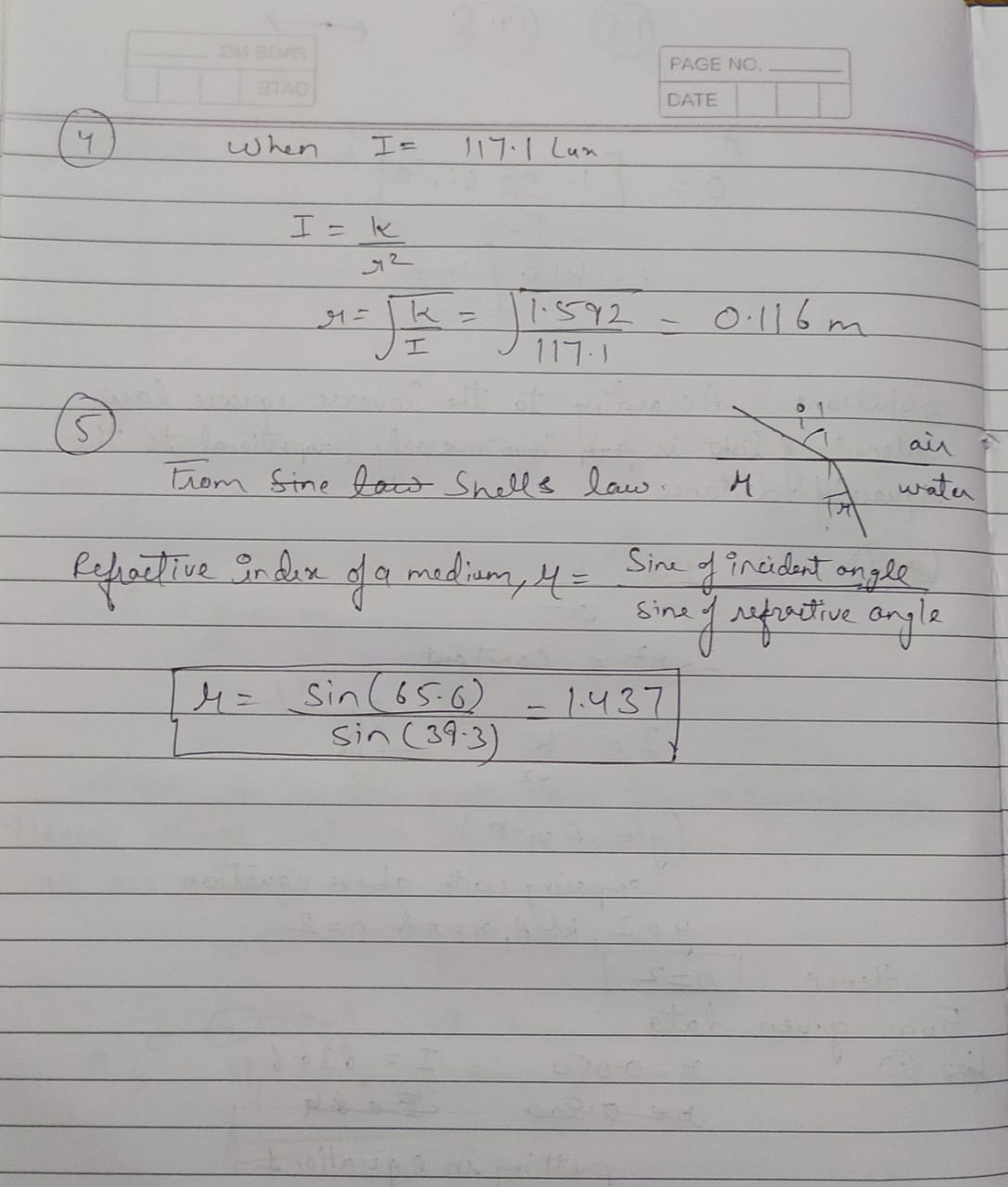#### Earn Coins

Coins can be redeemed for fabulous gifts.

Similar Homework Help Questions
• ### Partner: Date Name 11 Snell's Law Introduction When light passes from one material to another it ...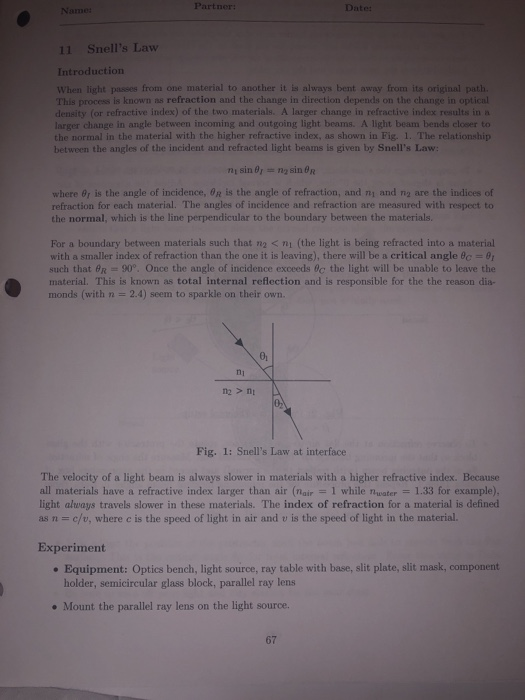Partner: Date Name 11 Snell's Law Introduction When light passes from one material to another it is always bent away from its original path. This process is known as refraction and the change in direction depends on the change in optical density (or refractive index) of the two materials. A larger change in refractive index results in a larger change in angle between incoming and outgoing light beams. A light beam bends closer to the normal in the material with...

• ### please do table 2. the top page is for website info. only. Part B Lab Go...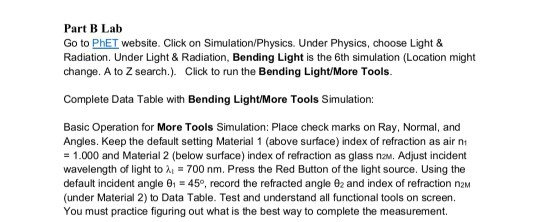please do table 2. the top page is for website info. only. Part B Lab Go to PhET website. Click on Simulation/Physics. Under Physics, choose Light & Radiation. Under Light & Radiation, Bending Light is the 6th simulation (Location might change. A to Z search.). Click to run the Bending Light/More Tools. Complete Data Table with Bending Light/More Tools Simulation: Basic Operation for More Tools Simulation: Place check marks on Ray, Normal, and Angles. Keep the default setting Material 1...

• ### Part B Lab Go to PhET website. Click on Simulation Physics. Under Physics, choose Light &...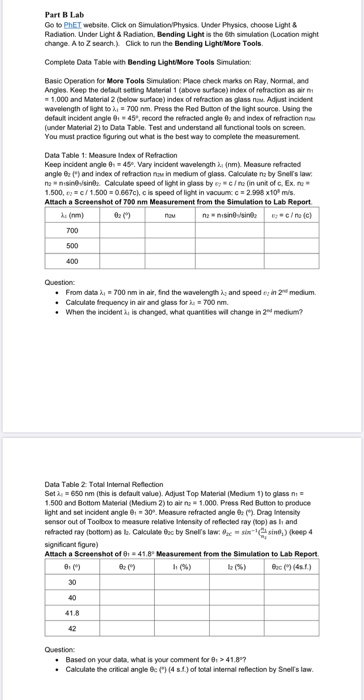Part B Lab Go to PhET website. Click on Simulation Physics. Under Physics, choose Light & Radiation. Under Light & Radiation, Bending Light is the 6th simulation (Location might change. A to Z search.) Click to run the Bending Light/More Tools. Complete Data Table with Bending Light/More Tools Simulation: Basic Operation for More Tools Simulation: Place check marks on Ray, Normal, and Angles. Keep the default setting Material 1 (above surface) index of refraction as air = 1.000 and Material...

• ### answer all questions. asurement of using the Sell remot Dispersion spectrum from a prism and me...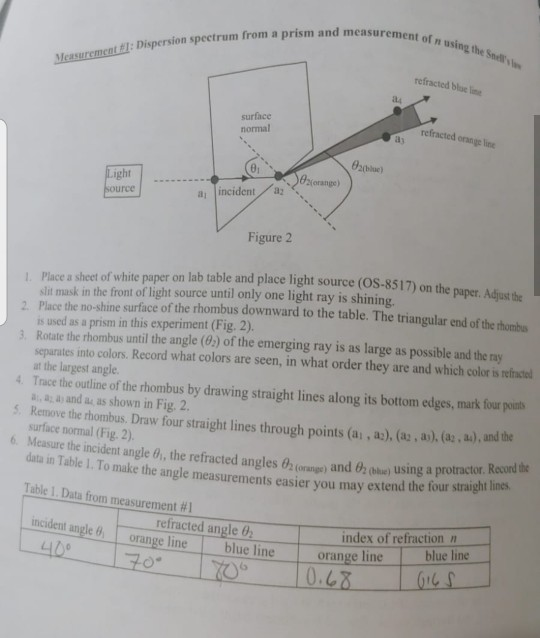answer all questions. asurement of using the Sell remot Dispersion spectrum from a prism and me refracted blue surface normal refracted online acted on 82 Light Source cope) a, incidenta: Figure 2 Place a sheet of white paper on lab table and place light source (OS-8517) on the slit mask in the front of light source until only one light ray is shining. the shine surface of the rhombus downward to the table. The triangular end of the is used...

• ### Ray Optics 1 Problem Statement Light strikes a 5.0-cm thick sheet of glass at an angle...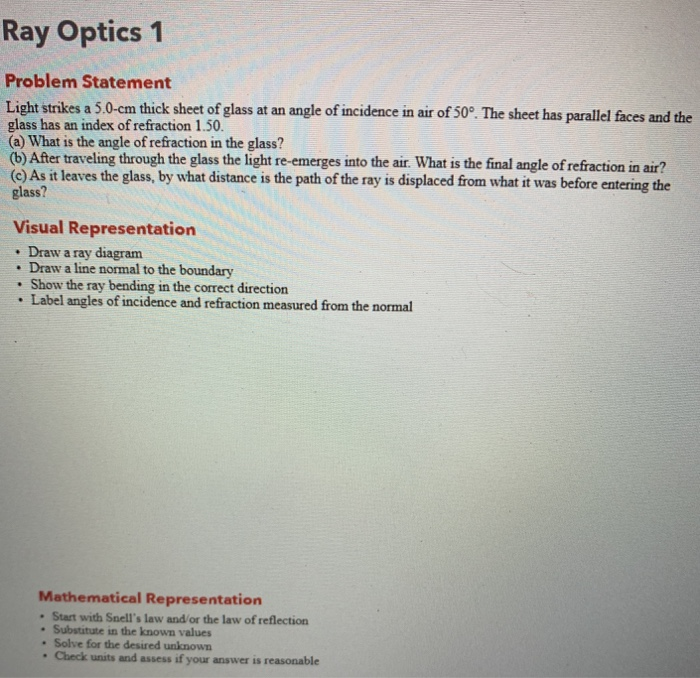Ray Optics 1 Problem Statement Light strikes a 5.0-cm thick sheet of glass at an angle of incidence in air of 50°. The sheet has parallel faces and the glass has an index of refraction 1.50. (a) What is the angle of refraction in the glass? (b) After traveling through the glass the light re-emerges into the air. What is the final angle of refraction in air? (c) As it leaves the glass, by what distance is the path of...

• ### Ray Optics 1 Problem Statement Light strikes a 5.0-cm thick sheet of glass at an angle...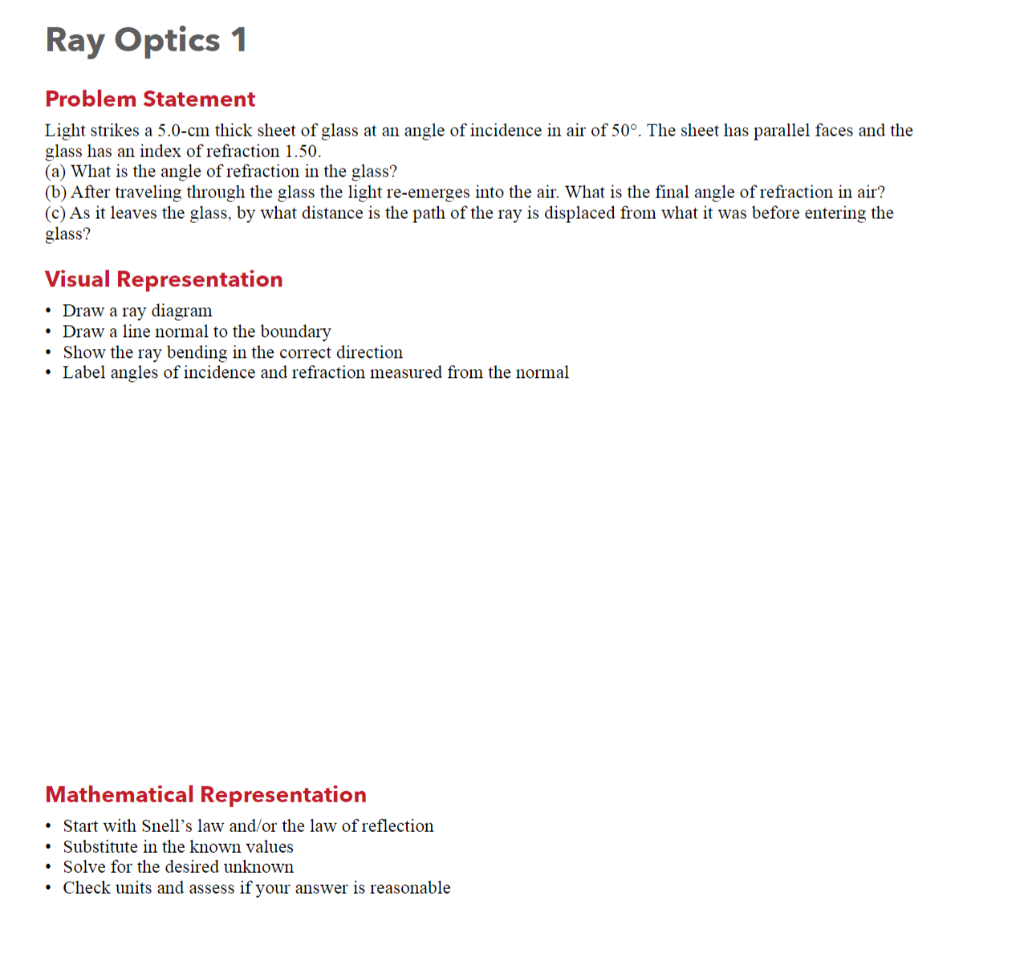Ray Optics 1 Problem Statement Light strikes a 5.0-cm thick sheet of glass at an angle of incidence in air of 50°. The sheet has parallel faces and the glass has an index of refraction 1.50. (a) What is the angle of refraction in the glass? (b) After traveling through the glass the light re-emerges into the air. What is the final angle of refraction in air? (c) As it leaves the glass, by what distance is the path of...

• ### Ray Optics 1 Problem Statement Light strikes a 5.0-cm thick sheet of glass at an angle...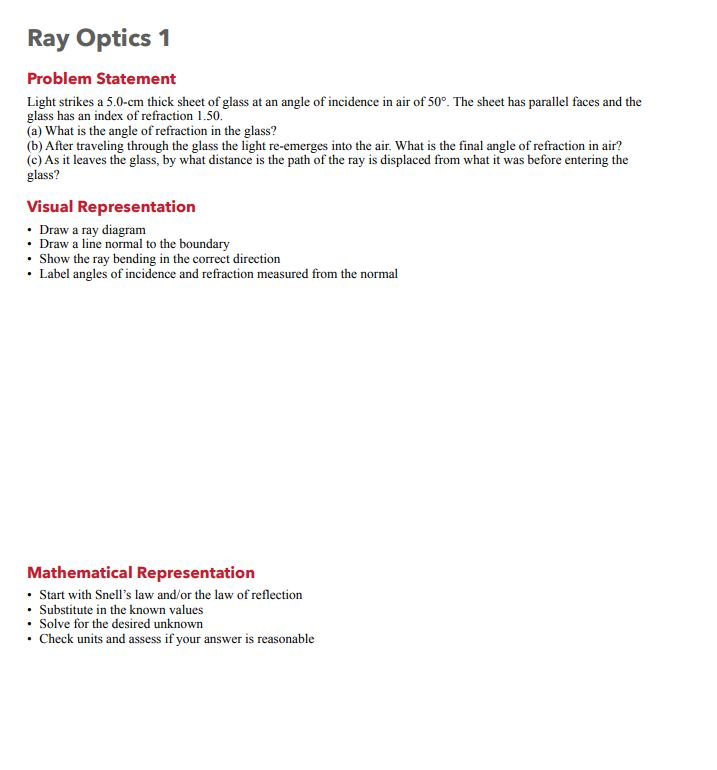Ray Optics 1 Problem Statement Light strikes a 5.0-cm thick sheet of glass at an angle of incidence in air of 50°. The sheet has parallel faces and the glass has an index of refraction 1.50. (a) What is the angle of refraction in the glass? (b) After traveling through the glass the light re-emerges into the air. What is the final angle of refraction in air? (c) As it leaves the glass, by what distance is the path of...

• ### Ray Optics 1 Problem Statement Light strikes a 5.0-cm thick sheet of glass at an angle...Ray Optics 1 Problem Statement Light strikes a 5.0-cm thick sheet of glass at an angle of incidence in air of 50°. The sheet has parallel faces and the glass has an index of refraction 1.50. (a) What is the angle of refraction in the glass? (b) After traveling through the glass the light re-emerges into the air. What is the final angle of refraction in air? (c) As it leaves the glass, by what distance is the path of...

• ### PLEASE HELP FILL OUT DATA TABLE 2 AND THE MISSING BLANKS IN DATA TABLE 1! let...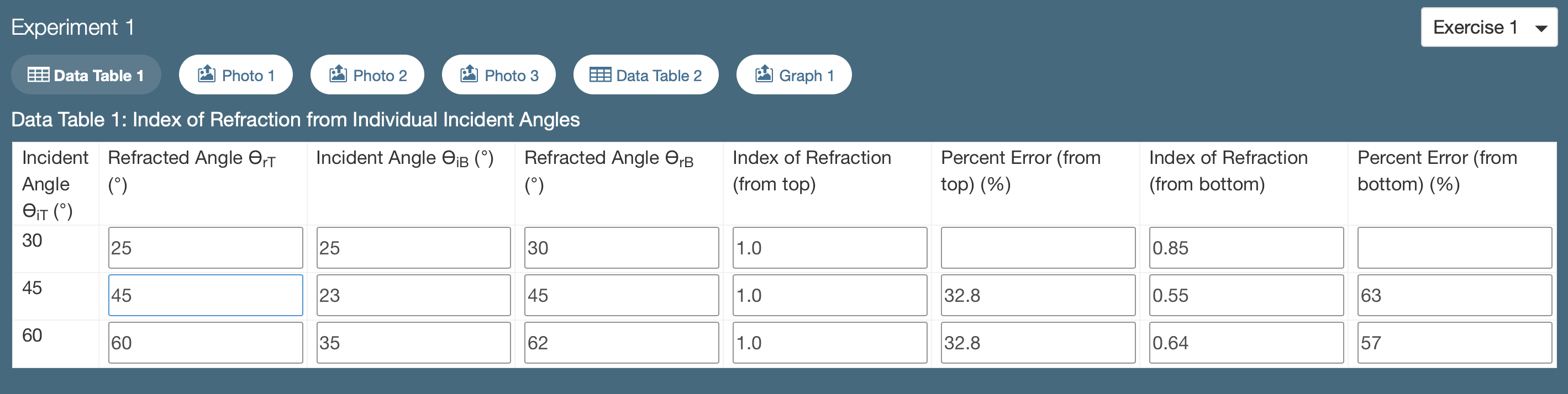PLEASE HELP FILL OUT DATA TABLE 2 AND THE MISSING BLANKS IN DATA TABLE 1! let me know if you have any questions / something needs to be fixed Experiment 1 Exercise 1 Data Table 1 Photo 1 Photo 2 Photo 3 Data Table 2 Graph 1 Data Table 1: Index of Refraction from Individual Incident Angles Incident Angle OiB (0) Refracted Angle OrB Index of Refraction (from top) Percent Error (from top) (%) Index of Refraction (from bottom) Percent...

• ### Part B Lab Go to PhET website. Click on Simulation/Physics. Under Physics, choose Light & Radiation....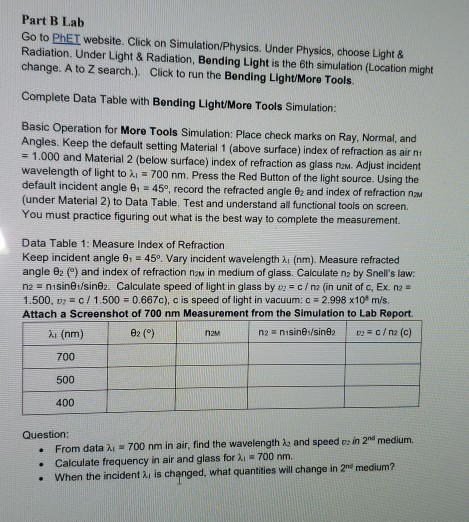Part B Lab Go to PhET website. Click on Simulation/Physics. Under Physics, choose Light & Radiation. Under Light & Radiation, Bending Light is the 6th simulation (Location might change. A to Z search.). Click to run the Bending Light/More Tools Complete Data Table with Bending Light/More Tools Simulation: Basic Operation for More Tools Simulation: Place check marks on Ray, Normal, and Angles. Keep the default setting Material 1 (above surface) index of refraction as air nt = 1.000 and Material...